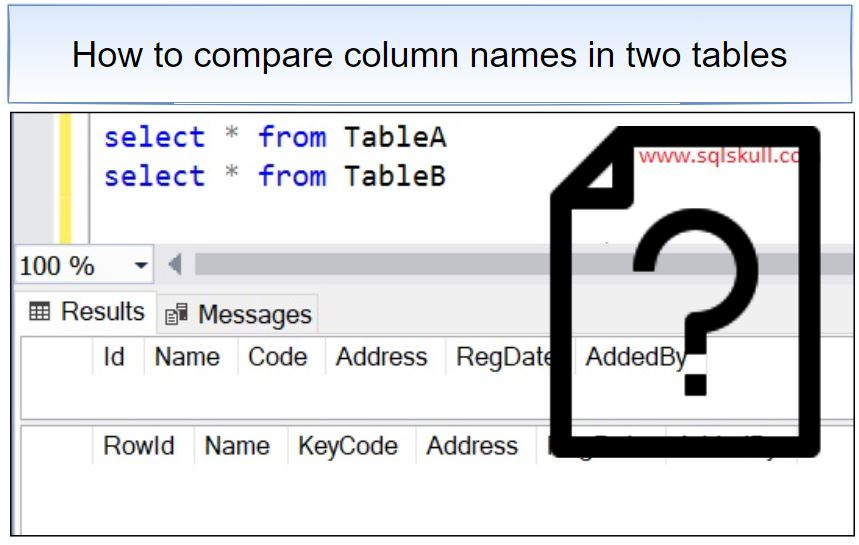Home » How to compare column names in two tables in SQL

# How to compare column names in two tables in SQLIn this article you will see how to compare column names in two table.

Assume that, you have two tables and you are asked to find out the columns which exists in both table with same name or column that exists in either of table.

Lets create a two sample table as shown below.

```Create table dbo.tableA
(
Id int,
Name varchar(100),
Code varchar(5),
RegDate datetime,
)

Create table dbo.tableB
(
RowId int,
Name varchar(100),
KeyCode varchar(5),
RegDate datetime,
)```

You can see, in both table few columns are having same name while few columns are different in both tables.Lets write a below SQL query to compare column name between these two tables.

```SELECT * into #tblA
FROM information_schema.columns
WHERE table_Schema ='dbo' and table_name = 'TableA' ;

SELECT * into #tblB
FROM information_schema.columns
WHERE table_Schema ='dbo' and table_name = 'TableB' ;

SELECT
COALESCE(A.Column_Name, B.Column_Name) AS [Column]
,CASE
WHEN (A.Column_Name IS NULL and B.Column_Name IS NOT NULL)
THEN 'Column - [' + B.Column_Name+ '] exists in Table - ['+ B.TABLE_NAME + '] Only'
WHEN (B.Column_Name IS NULL and A.Column_Name IS NOT NULL)
THEN 'Column - [' + A.Column_Name+ '] exist in Table - ['+ A.TABLE_NAME + '] Only'
WHEN A.Column_Name = B.Column_Name
THEN 'Column - [' + A.Column_Name + '] exists in both Table - ['+ A.TABLE_NAME + ' , ' + B.TABLE_NAME + ']'
END AS Remarks
FROM #tblA A
FULL JOIN #tblB B ON A.Column_Name = B.Column_Name;

drop table #tblA;
drop table #tblB;```

Lets execute the query and see the output.This site uses Akismet to reduce spam. Learn how your comment data is processed.